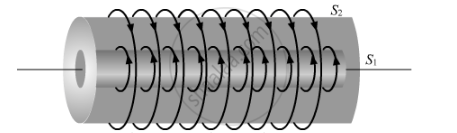# Obtain the Expression for Mutual Inductance of a Pair of Long Coaxial Solenoids - Physics

Obtain the expression for mutual inductance of a pair of long coaxial solenoids each of length l and radii r1 and r2 (r2 >> r1). Total number of turns in the two solenoids are N1 and N2, respectively.

#### SolutionSuppose a current i is passed through the inner solenoid S1.

A magnetic field B=μ0n1i is produced inside S1 , whereas the field outside it is zero.

The flux through each turn S2 is Bπr12=μ0n1iπr12

The total flux through all the turns in a length l of S2 is

ϕ=(μ0n1iπr12)n2l=(μ0n1n2πr12l)i

M=μ0n1n2πr12l

Concept: Solenoid and the Toroid - the Solenoid
Is there an error in this question or solution?
2013-2014 (March) All India Set 1

Share